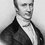# A calculus fallacy

We all are fimiler with the following well known results in calculus:-

(1) $\frac{d(x^n)}{dx}=nx^{n-1}$

(2) $\frac{d}{dx}(f_1(x)+f_2(x))=f'_1(x)+f'_2(x)$

Lets try to find the derivative of $x^2$ by using property (2):-

$x^2=\underbrace{x+x+x+...........+x}_\text{x times}$

Differentiating both sides of above equation:-

$\frac{d(x^2)}{dx}=\underbrace{1+1+1+......+1}_\text{x times}$

$\frac{d(x^2)}{dx}=x$

Which is horribly wrong,because $\frac{d(x^2)}{dx}=2x$Note by Aman Sharma
6 years, 7 months ago

This discussion board is a place to discuss our Daily Challenges and the math and science related to those challenges. Explanations are more than just a solution — they should explain the steps and thinking strategies that you used to obtain the solution. Comments should further the discussion of math and science.

When posting on Brilliant:

• Use the emojis to react to an explanation, whether you're congratulating a job well done , or just really confused .
• Ask specific questions about the challenge or the steps in somebody's explanation. Well-posed questions can add a lot to the discussion, but posting "I don't understand!" doesn't help anyone.
• Try to contribute something new to the discussion, whether it is an extension, generalization or other idea related to the challenge.

MarkdownAppears as
*italics* or _italics_ italics
**bold** or __bold__ bold
- bulleted- list
• bulleted
• list
1. numbered2. list
1. numbered
2. list
Note: you must add a full line of space before and after lists for them to show up correctly
paragraph 1paragraph 2

paragraph 1

paragraph 2

[example link](https://brilliant.org)example link
> This is a quote
This is a quote
    # I indented these lines
# 4 spaces, and now they show
# up as a code block.

print "hello world"
# I indented these lines
# 4 spaces, and now they show
# up as a code block.

print "hello world"
MathAppears as
Remember to wrap math in $$ ... $$ or $ ... $ to ensure proper formatting.
2 \times 3 $2 \times 3$
2^{34} $2^{34}$
a_{i-1} $a_{i-1}$
\frac{2}{3} $\frac{2}{3}$
\sqrt{2} $\sqrt{2}$
\sum_{i=1}^3 $\sum_{i=1}^3$
\sin \theta $\sin \theta$
\boxed{123} $\boxed{123}$

Sort by:

A function $f$ is not differentiable if it is not continuous. While your reasoning is correct for $x \in \mathbb{N}$, it does not apply to $x \in \mathbb{R} \setminus \mathbb{N}$; hence, $f$ is not continuous and therefore not differentiable.

- 6 years, 6 months ago

Try writing $\pi ^2$ as $\pi$ a $\pi$ number of times. Or more simply $3.5^2$ as a sum of $3.5 \text{ } 3.5 '$s . To make it more obvious just write $25$ as $5$ added $5$ times.

- 6 years, 7 months ago

When you are defining x^2=x+x+..+x(x times), you are tacitly defining the domain of f(x) to be N, the set of natural numbers. However, f(x) cannot be differentiable in the domain of N. This is because, if a function f defined from a domain D (a subset of R, the set of reals) to R (the set of reals) has to be differentiable at a real point c, then a necessary criterion is that c has to be a limit point of D and c has to be an element of D itself. In other words, c has to be a member of D such that every arbitrarily small neighbourhood of c has an element of D other than c. But no natural number is a cluster point of the set N. Here lies the fallacy. (last modified 1 hour ago )

very nicely explained. Thanks a ton mate.

- 5 years, 11 months ago

- 5 years, 11 months ago

In differentiation, we are concerned with the ratio of change of one quantity at a particular instant with respect to another.

- 6 years, 7 months ago